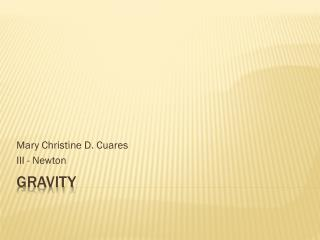Download PresentationGravity

# Gravity - PowerPoint PPT PresentationDownload Presentation## Gravity

- - - - - - - - - - - - - - - - - - - - - - - - - - - E N D - - - - - - - - - - - - - - - - - - - - - - - - - - -
##### Presentation Transcript

1. Mary Christine D. Cuares III - Newton Gravity

2. Is a fundamental force in the sense that it cannot be explained in terms of any other force. Four Fundamental Forces: Gravitational, Electromagnetic, Weak Nuclear, Strong Nuclear Force The weak and strong forces have very short ranges and act within atomic nuclei. Defining Gravity

3. The weak force is closely related to the electromagnetic force, and the strong force seems to be related to both of them. Electromagnetic forces, which like are unlimited in range, act between electrically charged particles and govern the structures and behavior of atoms, molecules, solids amd liquids.

4. When an object touches another, an electromagnetic force is what each exerts on the other.

5. How much force holds a 220-pound man in his seat in newtons? Answer Given: Mass of the man m 220 pounds Find: Force that holds him in his seat in N Solution: The force that holds the man in his seat is numerically equal, but opposite in direction to his weight. Example 1

6. Since the force (and weight) must be in newtons, we must first convert pounds to kg. 1kg 220 pounds x ------------------------- = 100 kg 2.2 ;pounds Applying the equation for weight w = mg = 100 kg (9.8m/s2) = 980 N The force 980N directed upward, as opposed too his weight, which is directed downward.

7. Your weight on Earth, or the force that pulls you toward the ground, is the pull of Earth’s gravity on you. Weight = mass x acceleration due to gravity or w = mg Weight = Newton Mass = Kilogram Acceleration due to gravity = 9.8 m/s2 How Can Your Weight Change?

8. From the previous example, how much does he weigh on the moon if the gravity is 1/6th of the earth? What acceleration due to gravity on the moon? Giiven: Weight on the earth Wearth 980 N Acceleration(gravity) gearth 9.8 m/s2 Gravity on the moon 1/6th of the earth’s gravity Example 2

9. Find: • Weight on the moon (Wmoon) • Acceleration due to gravity on the moon Solution: • Since your weight on the earth is 980 N, and the gravity of the moon is 1/6th that of the earth, then just divided your weight on the earth by 6. WEIGHTmoon = 980 N / 6 = 163 N

10. To solve for gmoon, again, just divide gearth by 6. gmoon= 9.8 m/s2/ 6 = 1.6 m/s2

11. Two basic ways to feel weightless: • You can be far enough away from all large objects in the universe that the force of gravity due to them cannot be felt. • You can be in free fall. • When you are falling toward the Earth with an acceleration of 9.8 m/s2, you feel weightless. • Free fall is when the force of gravity is busy accelerating you and there is no force left over to keep you pushed into your seat. Weightlessness